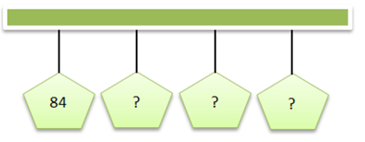Mathematics
Easy

Question

# The house numbers on the street follow the rule "Add 4." If the pattern continues, what are the next three house numbers?## 87, 90, 93    85, 89, 93    88, 92, 96    86, 90, 94Hint:

## The correct answer is: 88, 92, 96

### The house number of first house is 84.It means the first term of number sequence is 84.The rule used is to add four.We have to add 4 to the current term, to get the next term. We have to find three terms.84 + 4 = 8888 + 4 = 9292 + 4 = 96So, the complete sequence of house numbers will be 84, 88, 92, 96.Therefore, the next terms are 88, 92, 96

For such questions, we should know the basic operations. When the rule used to write the pattern is not mentioned, we have to use all four operations to check the pattern. The operations are addition, subtraction, division, and multiplication.

### Related Questions to study#### With Turito Foundation.#### Get an Expert Advice From Turito.